The “forecastHybrid” package provides functions to build composite models using multiple individual component models from the “forecast” package. These hybridModel objects can then be manipulated with many of the familiar functions from the “forecast” and “stats” packages including forecast(), plot(), accuracy(), residuals(), and fitted().

# Installation

The stable release of the package is hosted on CRAN and can be installed as usual.

install.packages("forecastHybrid")

The latest development version can be installed using the “devtools” package.

devtools::install_github("ellisp/forecastHybrid/pkg")

Version updates to CRAN will be published frequently after new features are implemented, so the development version is not recommended unless you plan to modify the code.

# Basic usage

library(forecastHybrid)

## Quick start

If you don’t have time to read the whole guide and want to get started immediatly with sane default settings to forecast the USAccDeaths timeseries, run the following:

quickModel <- hybridModel(USAccDeaths)
## Fitting the auto.arima model
## Fitting the ets model
## Fitting the thetam model
## Fitting the nnetar model
## Fitting the stlm model
## Fitting the tbats model
forecast(quickModel)
##          Point Forecast    Lo 80     Hi 80    Lo 95     Hi 95
## Jan 1979       8345.710 7924.712  8974.280 7699.151  9248.352
## Feb 1979       7539.386 6738.653  8115.771 6404.285  8507.340
## Mar 1979       8239.352 7203.760  8842.792 6883.533  9122.377
## Apr 1979       8531.709 7618.560  9194.629 7310.117  9500.477
## May 1979       9343.289 8166.269 10112.349 7868.037 10442.376
## Jun 1979       9763.362 8506.733 10525.745 8199.822 10878.297
## Jul 1979      10690.864 9144.638 11613.448 8744.951 11987.171
## Aug 1979       9975.123 9042.183 10830.331 8771.135 11224.086
## Sep 1979       8988.239 8332.971  9944.791 7972.309 10357.609
## Oct 1979       9241.620 8239.467 10198.510 7966.788 10629.548
## Nov 1979       8755.561 8003.768  9732.246 7589.183 10180.765
## Dec 1979       9106.393 8292.019 10255.628 7938.606 10720.971
## Jan 1980       8356.204 7495.330  9496.297 7033.420 10011.749
## Feb 1980       7575.450 6687.306  8749.398 6141.503  9295.201
## Mar 1980       8262.126 6991.917  9586.522 6699.833 10161.075
## Apr 1980       8552.909 7384.111  9940.466 7064.387 10542.396
## May 1980       9350.977 7838.925 10861.976 7482.906 11490.092
## Jun 1980       9755.246 8121.405 11280.306 7797.162 11933.559
## Jul 1980      10671.807 8556.176 12373.743 8128.319 13051.201
## Aug 1980       9944.684 8612.255 11596.927 8248.308 12297.755
## Sep 1980       8975.446 7984.866 10718.099 7261.423 11441.542
## Oct 1980       9231.879 7963.456 10978.824 7417.369 11724.196
## Nov 1980       8750.182 7623.215 10519.779 6856.540 11286.453
## Dec 1980       9099.520 7892.976 11050.531 7288.262 11837.932
plot(forecast(quickModel), main = "Forecast from auto.arima, ets, thetam, nnetar, stlm, and tbats model")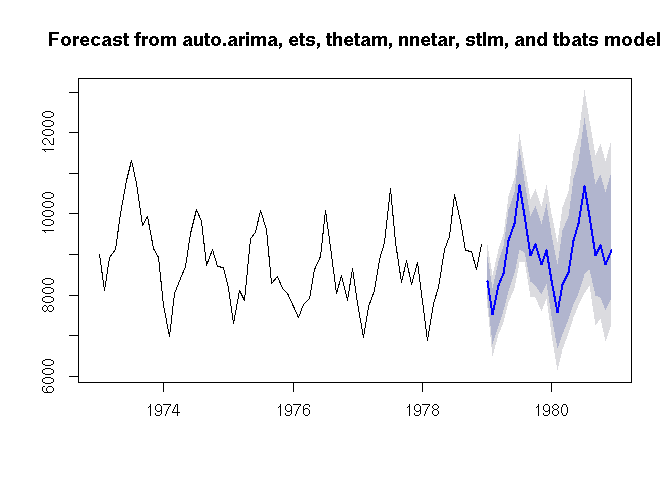## Fitting a model

The workhorse function of the package is hybridModel(), a function that combines several component models from the “forecast” package. At a minimum, the user must supply a ts or numeric vector for y. In this case, the ensemble will include all six component models: auto.arima(), ets(), thetam(), nnetar(), stlm(), and tbats(). To instead use only a subset of these models, pass a character string to the models argument with the first letter of each model to include. For example, to build an ensemble model on a simulated dataset with auto.arima(), ets(), and tbats() components, run

# Build a hybrid forecast on a simulated dataset using auto.arima, ets, and tbats models.
# Each model is given equal weight
set.seed(12345)
series <- ts(rnorm(18), f = 2)
hm1 <- hybridModel(y = series, models = "aet", weights = "equal")
## Fitting the auto.arima model
## Fitting the ets model
## Fitting the tbats model

The individual component models are stored inside the hybridModel objects and can viewed in their respective slots, and all the regular methods from the “forecast” package could be applied to these individual component models.

# View the individual models
hm1$auto.arima ## Series: structure(c(0.585528817843856, 0.709466017509524, -0.109303314681054, -0.453497173462763, 0.605887455840394, -1.81795596770373, 0.630098551068391, -0.276184105225216, -0.284159743943371, -0.919322002474128, -0.116247806352002, 1.81731204370422, 0.370627864257954, 0.520216457554957, -0.750531994502331, 0.816899839520583, -0.886357521243213, -0.331577589942552), .Tsp = c(1, 9.5, 2), class = "ts") ## ARIMA(0,0,0)(0,1,0) ## ## sigma^2 estimated as 1.247: log likelihood=-24.47 ## AIC=50.94 AICc=51.23 BIC=51.71 # See forecasts from the auto.arima model plot(forecast(hm1$auto.arima))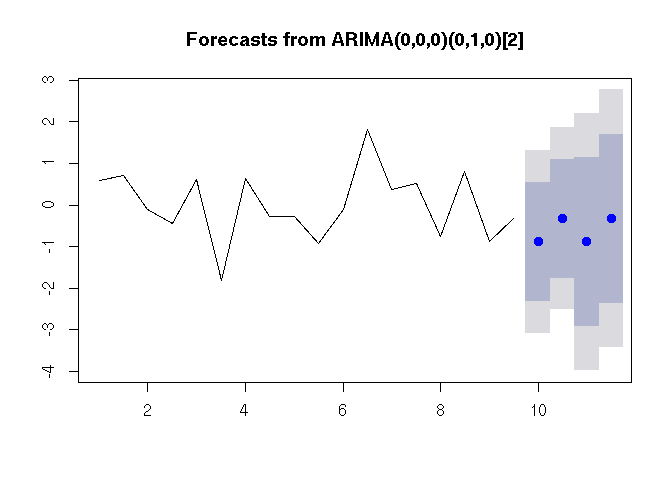### Model diagnostics

The hybridModel() function produces an S3 object of class forecastHybrid.

class(hm1) 
##  "hybridModel"
is.hybridModel(hm1)
##  TRUE

The print() and summary() methods print information about the ensemble model including the weights assigned to each individual component model.

print(hm1) 
## Hybrid forecast model comprised of the following models: auto.arima, ets, tbats
## ############
## auto.arima with weight 0.333
## ############
## ets with weight 0.333
## ############
## tbats with weight 0.333
summary(hm1)
##            Length Class  Mode
## auto.arima 18     ARIMA  list
## ets        19     ets    list
## tbats      21     bats   list
## weights     3     -none- numeric
## frequency   1     -none- numeric
## x          18     ts     numeric
## xreg        1     -none- list
## models      3     -none- character
## fitted     18     ts     numeric
## residuals  18     ts     numeric

Two types of plots can be created for the created ensemble model: either a plot showing the actual and fitted value of each component model on the data or individual plots of the component models as created by their regular S3 plot() methods. Note that a plot() method does not exist in the “forecast” package for objects generated with stlm(), so this component model will be ignored when type = "models", but the other component models will be plotted regardless.

plot(quickModel, type = "fit")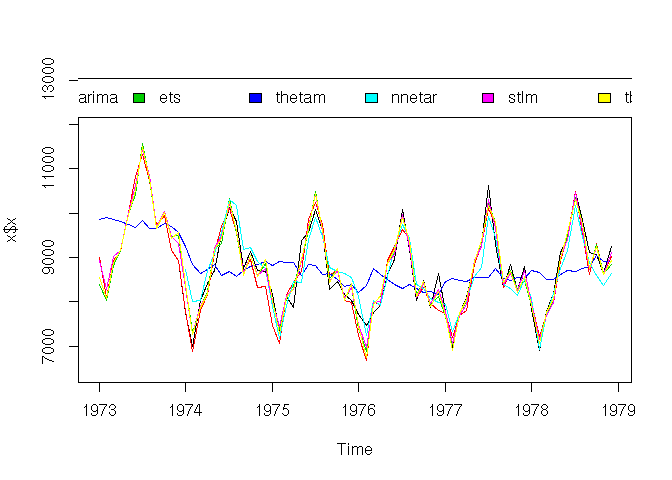plot(quickModel, type = "models")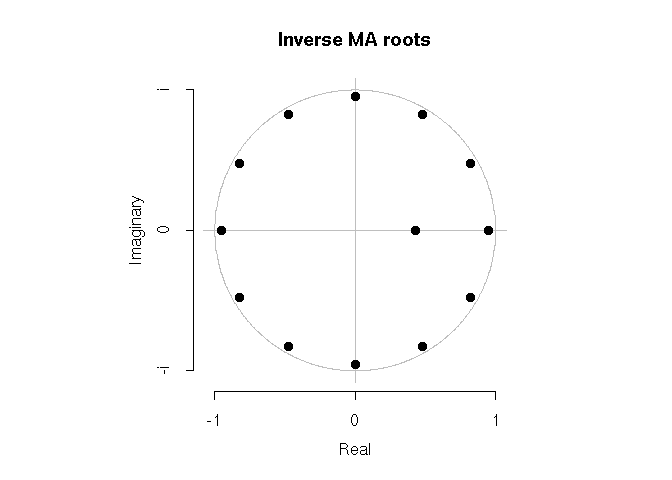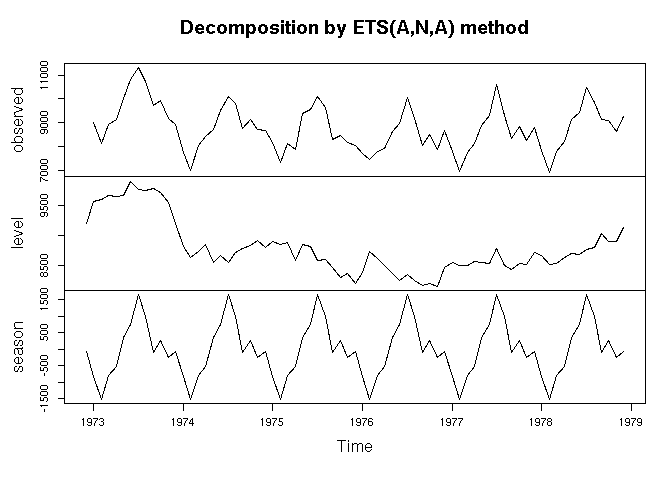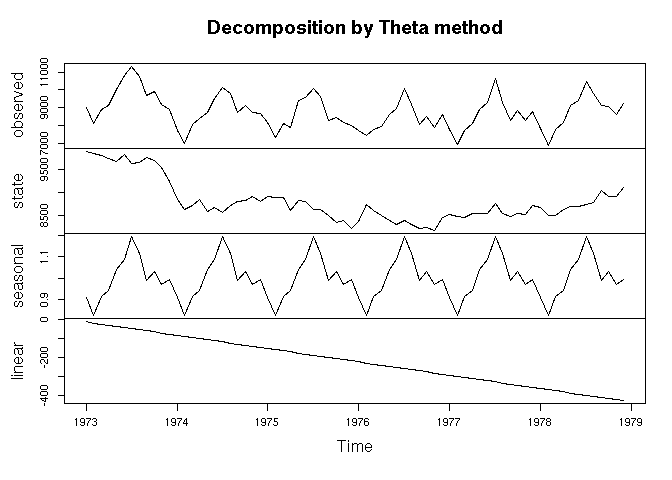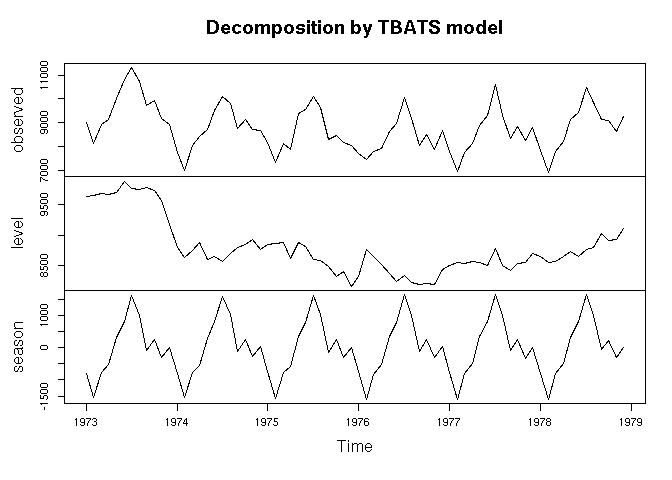Since version 0.4.0, ggplot graphs are available. Note, however, that the nnetar, and tbats models do not have ggplot::autoplot() methods, so these are not plotted.

plot(quickModel, type = "fit", ggplot = TRUE)
## Warning: attributes are not identical across measure variables; they will
## be dropped
## Warning: Removed 12 rows containing missing values (geom_path).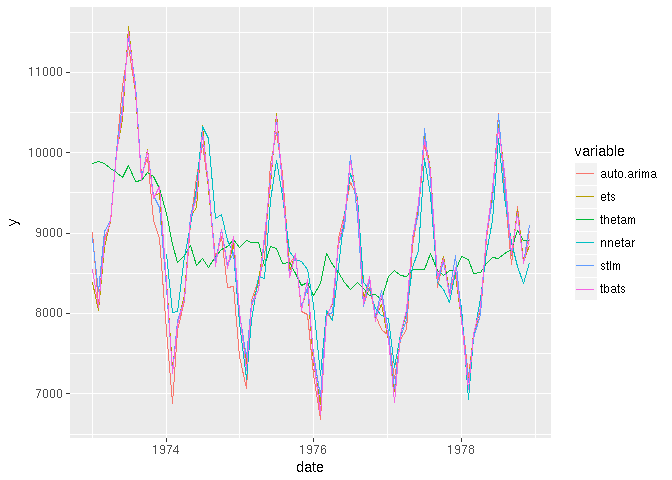plot(quickModel, type = "models", ggplot = TRUE)

By default each component model is given equal weight in the final ensemble. Empirically this has been shown to give good performance in ensembles [see @Armstrong2001], but alternative combination methods are available: the inverse root mean square error (RMSE), inverse mean absolute error (MAE), and inverse mean absolute scaled error (MASE). To apply one of these weighting schemes of the component models, pass this value to the errorMethod argument and pass either "insample.errors" or "cv.errors" to the weights argument.

hm2 <- hybridModel(series, weights = "insample.errors", errorMethod = "MASE", models = "aenst")
## Warning in hybridModel(series, weights = "insample.errors", errorMethod =
## "MASE", : Using insample.error weights is not recommended for accuracy and
## may be deprecated in the future.
## Fitting the auto.arima model
## Fitting the ets model
## Fitting the nnetar model
## Fitting the stlm model
## Fitting the tbats model
hm2 
## Hybrid forecast model comprised of the following models: auto.arima, ets, nnetar, stlm, tbats
## ############
## auto.arima with weight 0.135
## ############
## ets with weight 0.163
## ############
## nnetar with weight 0.305
## ############
## stlm with weight 0.204
## ############
## tbats with weight 0.193

After the model is fit, these weights are stored in the weights attribute of the model. The user can view and manipulated these weights after the fit is complete. Note that the hybridModel() function automatically scales weights to sum to one, so a user should similar scale the weights to ensure the forecasts remain unbiased. Furthermore, the vector that replaces weights must retain names specifying the component model it corresponds to since weights are not assigned by position but rather by component name. Similarly, indiviudal components may also be replaced

hm2$weights  ## auto.arima ets nnetar stlm tbats ## 0.1354514 0.1629405 0.3046499 0.2041276 0.1928306 newWeights <- c(0.1, 0.2, 0.3, 0.1, 0.3) names(newWeights) <- c("auto.arima", "ets", "nnetar", "stlm", "tbats") hm2$weights <- newWeights
hm2
## Hybrid forecast model comprised of the following models: auto.arima, ets, nnetar, stlm, tbats
## ############
## auto.arima with weight 0.1
## ############
## ets with weight 0.2
## ############
## nnetar with weight 0.3
## ############
## stlm with weight 0.1
## ############
## tbats with weight 0.3
hm2$weights <- 0.2 hm2$weights <- 0.1
hm2
## Hybrid forecast model comprised of the following models: auto.arima, ets, nnetar, stlm, tbats
## ############
## auto.arima with weight 0.2
## ############
## ets with weight 0.1
## ############
## nnetar with weight 0.3
## ############
## stlm with weight 0.1
## ############
## tbats with weight 0.3

This hybridModel S3 object can be manipulated with the same familiar interface from the “forecast” package, including S3 generic functions such as accuracy, forecast, fitted, and residuals.

# View the first 10 fitted values and residuals
head(fitted(hm1))
## Time Series:
## Start = c(1, 1)
## End = c(3, 2)
## Frequency = 2
##   0.20769208  0.24714602  0.20382787  0.24556301 -0.02580335 -0.14243955
head(residuals(hm1))
## Time Series:
## Start = c(1, 1)
## End = c(3, 2)
## Frequency = 2
##   0.20769208  0.24714602  0.20382787  0.24556301 -0.02580335 -0.14243955

In-sample errors and various accuracy measure can be extracted with the accuracy method. The “forecastHybrid” package creates an S3 generic from the accuracy method in the “forecast” package, so accuracy will continue to function as normal with objects from the “forecast” package, but now special functionality is created for hybridModel objects. To view the in-sample accuracy for the entire ensemble, a simple call can be made.

accuracy(hm1) 
##                   ME      RMSE       MAE     MPE     MAPE      ACF1
## Test set -0.05482306 0.8153455 0.6475946 93.3401 108.1688 -0.111188
##          Theil's U
## Test set  1.088137

In addition to retrieving the ensemble’s accuracy, the individual component models’ accuracies can be easily viewed by using the individual = TRUE argument.

accuracy(hm1, individual = TRUE) 
## $auto.arima ## ME RMSE MAE MPE MAPE MASE ## Training set -0.1395353 1.052906 0.8029324 75.46075 181.2409 0.8889685 ## ACF1 ## Training set 0.01339733 ## ##$ets
##                        ME      RMSE       MAE      MPE     MAPE     MASE
## Training set -0.000337709 0.8160167 0.6674726 100.7733 100.7733 0.738994
##                    ACF1
## Training set -0.2202415
##
## $tbats ## ME RMSE MAE MPE MAPE MASE ## Training set -0.02459618 0.812366 0.6684029 103.7863 103.7863 0.6244447 ## ACF1 ## Training set -0.2201724 ## Forecasting Now’s let’s forecast future values. The forecast() function produce an S3 class forecast object for the next 48 periods from the ensemble model. hForecast <- forecast(hm1, h = 48)  Now plot the forecast for the next 48 periods. The prediction intervals are preserved from the individual component models and currently use the most extreme value from an individual model, producing a conservative estimate for the ensemble’s performance. plot(hForecast)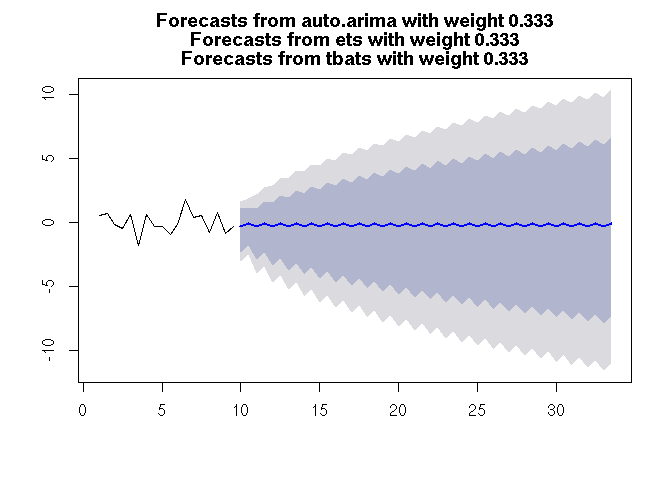# Advanced usage The package aims to make fitting ensembles easy and quick, but it still allows advanced tuning of all the parameters available in the “forecast” package. This is possible through usage of the a.args, e.args, n.args, s.args, and t.args lists. These optional list arguments may be applied to one, none, all, or any combination of the included individual component models. Consult the documentation in the “forecast” package for acceptable arguments to pass in the auto.arima, ets, nnetar, stlm, and tbats functions. hm3 <- hybridModel(y = series, models = "aefnst", a.args = list(max.p = 12, max.q = 12, approximation = FALSE), n.args = list(repeats = 50), s.args = list(robust = TRUE), t.args = list(use.arma.errors = FALSE))  ## Fitting the auto.arima model ## Fitting the ets model ## Fitting the thetam model ## Fitting the nnetar model ## Fitting the stlm model ## Fitting the tbats model Since the lambda argument is shared between most of the models in the “forecast” framework, it is included as a special paramemeter that can be used to set the Box-Cox transform in all models instead of settings this individually. For example, hm4 <- hybridModel(y = wineind, models = "ae", lambda = 0.15) ## Fitting the auto.arima model ## Fitting the ets model hm4$auto.arima$lambda  ##  0.15 ## attr(,"biasadj") ##  FALSE hm4$ets$lambda ##  0.15 ## attr(,"biasadj") ##  FALSE Users can still apply the lambda argument through the tuning lists, but in this case the list-supplied argument overwrites the default used across all models. Compare the following two results. hm5 <- hybridModel(y = USAccDeaths, models = "aens", lambda = 0.2, a.args = list(lambda = 0.5), n.args = list(lambda = 0.6))  ## Fitting the auto.arima model ## Fitting the ets model ## Fitting the nnetar model ## Fitting the stlm model hm5$auto.arima$lambda ##  0.5 ## attr(,"biasadj") ##  FALSE hm5$ets$lambda ##  0.2 ## attr(,"biasadj") ##  FALSE hm5$nnetar$lambda ##  0.6 hm5$stlm$lambda ##  0.2 ## attr(,"biasadj") ##  FALSE Note that lambda has no impact on thetam models, and that there is no f.args argument to provide parguments to thetam. Following forecast::thetaf on which thetam is based, there are no such arguments; it always runs with the defaults. Covariates can also be supplied to auto.arima and nnetar models as is done in the “forecast” package. To do this, utilize the a.args and n.args lists. Note that the xreg may also be passed to a stlm model, but only when method = "arima" instead of the default method = "ets". Unlike the usage in the “forecast” package, the xreg argument should be passed as a dataframe, not a matrix. The stlm models require that the input series will be seasonal, so in the example below we will convert the input data to a ts object. If a xreg is used in training, it must also be supplied to the forecast() function in the xreg argument. Note that if the number of rows in the xreg to be used for the forecast does not match the supplied h forecast horizon, the function will overwrite h with the number of rows in xreg and issue a warning. # Use the beaver1 dataset with the variable "activ" as a covariate and "temp" as the timeseries # Divice this into a train and test set trainSet <- beaver1[1:100, ] testSet <- beaver1[-(1:100), ] trainXreg <- data.frame(trainSet$activ)
testXreg <- data.frame(testSet$activ) # Create the model beaverhm <- hybridModel(ts(trainSet$temp, f = 6),
models = "aenst",
a.args = list(xreg = trainXreg),
n.args = list(xreg = trainXreg),
s.args = list(xreg = trainXreg, method = "arima"))
## Fitting the auto.arima model
## Fitting the ets model
## Fitting the nnetar model
## Fitting the stlm model
## Fitting the tbats model
# Forecast future values
beaverfc <- forecast(beaverhm, xreg = testXreg)

# View the accuracy of the model
accuracy(beaverfc, testSet$temp) ## ME RMSE MAE MPE MAPE ## Training set 0.0005986523 0.07667039 0.05179402 0.001264137 0.1402911 ## Test set 0.0835995737 0.11214383 0.09329672 0.226223710 0.2526417 ## MASE ACF1 ## Training set 0.7852386 0.0162318 ## Test set 1.4144526 NA ## Cross Validation It can be useful to perform cross validation on a forecasting model to estimate a model’s out-of-sample forecasting performance. The cvts() function allows us to do this on arbitrary functions. We could do this as part of a model selection procedure to determine which models to include in our call to hybridModel() or merely to understand how well we expect to forecast the series during unobserved windows. For example, let’s perform cross validation for a stlm() model and a naive() model on the woolyrnq timeseries. The most important cvts() arguments that commonly need adjusting are rolling (if TRUE, the model will always be fit on a fixed windowSize instead of growing by one new observation for each new model fit during cross validation), windowSize (starting length of time series to fit a model), and maxHorizon (the forecast horizon for predictions from each model). Since a naive forecast is a good baseline that any decent model should surpass, let’s see how the stlm() model compares. stlmMod <- cvts(woolyrnq, FUN = stlm, windowSize = 100, maxHorizon = 8) naiveMod <- cvts(woolyrnq, FUN = naive, windowSize = 100, maxHorizon = 8) accuracy(stlmMod) ## ME RMSE MAE ## Forecast Horizon 1 191.13714 339.82149 280.97196 ## Forecast Horizon 2 -194.44269 345.31349 285.36546 ## Forecast Horizon 3 -173.62950 192.63326 173.62950 ## Forecast Horizon 4 -87.48572 124.76727 88.95572 ## Forecast Horizon 5 -80.86286 95.58736 80.86286 ## Forecast Horizon 6 -75.94269 294.81456 284.86546 ## Forecast Horizon 7 51.37050 69.33831 51.37050 ## Forecast Horizon 8 -67.48572 145.54690 128.95572 accuracy(naiveMod) ## ME RMSE MAE ## Forecast Horizon 1 -768.5 802.0365 768.5 ## Forecast Horizon 2 -229.0 324.5628 230.0 ## Forecast Horizon 3 142.5 184.0611 142.5 ## Forecast Horizon 4 -88.0 124.4508 88.0 ## Forecast Horizon 5 -1040.5 1040.5001 1040.5 ## Forecast Horizon 6 -110.5 254.7165 229.5 ## Forecast Horizon 7 367.5 367.7479 367.5 ## Forecast Horizon 8 -68.0 144.9414 128.0 We see from looking at the accuracy measure–in particular the smaller RMSE and MAE–the stlm() model unsurprisingly performs better and will likely give us better future forecasts. We also notice that the apparent edge over the naive forecast tends to diminish or even disappear for longer forecast horizons, and a look at the original timeseries makes this result obvious: this timeseries lacks an obvious trend and is a relatively difficult timeseries to forecast past a fewer seasonal periods, so the naive model will not perform relatively poorly. plot(woolyrnq)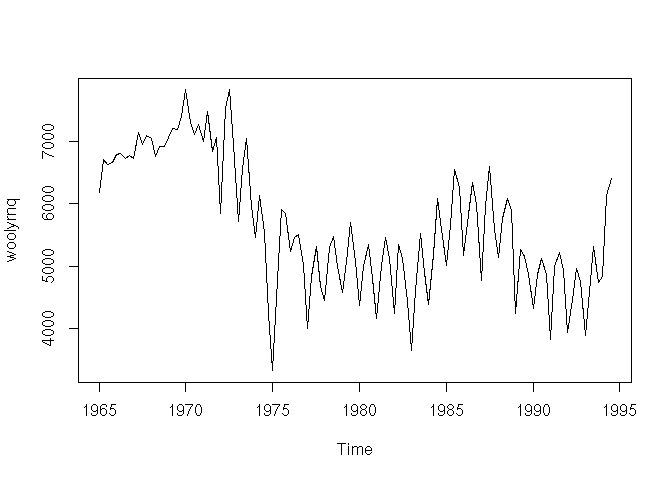We can also use custom functions, for example fcast() from the “GMDH” package. We must be very careful that our custom forecast function still produces an expected “forecast” S3 class object and that the ts object start, end, and frequency properties are preserved. library(GMDH) GMDHForecast <- function(x, h){ fc <- GMDH::fcast(x, f.number = h) # GMDH doesn't produce a ts object with correct attributes, so we build it end <- tsp(x) freq <- frequency(x) # Set the correct start, end, and frequency for the ts forecast object tsProperties <- c(end + 1 / freq, end + h / freq, freq) tsp(fc$mean) <- tsProperties
tsp(fc$upper) <- tsProperties tsp(fc$lower) <- tsProperties
class(fc) <- "forecast"
return(fc)
}
series <- subset(woolyrnq, end = 12)
gmdhcv <- cvts(series, FCFUN = GMDHForecast, windowSize = 10, maxHorizon = 1)

As a final example, suppose we foolish want to implement our own version of naive() for performing cross validation. The FUN and FCFUN could then look like

customMod <- function(x){
result <- list()
result$series <- x result$last <- tail(x, n = 1)
class(result) <- "customMod"
return(result)
}
forecast.customMod <- function(x, h = 12){
result <- list()
result$model <- x result$mean <- rep(x\$last, h)
class(result) <- "forecast"
return(result)
}
series <- subset(AirPassengers, end = 94)

cvobj <- cvts(series, FUN = customMod, FCFUN = forecast.customMod)

## Cross Validation Weights

Previously we explored fitting hybridModel() objects with weights = "equal" or weights = "insample.errors, but we can now leverage the process conducted in cvts() to select the appropriate weights inteligently based on the expected out-of-sample forecast accuracy of each component model. While this is the methodologically-sound weight procedure, it also comes at significant computational cost since the cross validation procedure necessitates fitting each model several times for each cross validation fold in addition to the final fit on the whole dataset. Fortunately this process can be conducted in parallel if multiple cores are available. Some of the arguments explained above in cvts() such as windowSize and the cvHorizon can also be controlled here.

cvMod <- hybridModel(woolyrnq, models = "ns", weights = "cv.errors", windowSize = 100, cvHorizon = 8, num.cores = 4)
cvMod

`